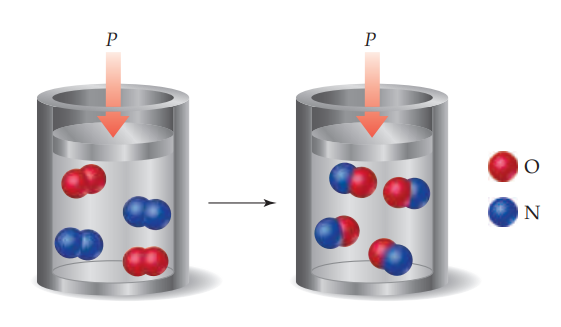×
Get Full Access to Chemistry: The Central Science - 14 Edition - Chapter 5 - Problem 5.10
Get Full Access to Chemistry: The Central Science - 14 Edition - Chapter 5 - Problem 5.10

×

# ?The gas-phase reaction shown, between $$\mathrm{N}_{2} \text { and } \mathrm{O}_{2}$$, was run in an apparatus designed to maintain a constant pressurISBN: 9780134414232 1274

## Solution for problem 5.10 Chapter 5

Chemistry: The Central Science | 14th Edition

• Textbook Solutions
• 2901 Step-by-step solutions solved by professors and subject experts
• Get 24/7 help from StudySoup virtual teaching assistantsChemistry: The Central Science | 14th Edition

4 5 1 422 Reviews
15
4
Problem 5.10

The gas-phase reaction shown, between $$\mathrm{N}_{2} \text { and } \mathrm{O}_{2}$$, was run in an apparatus designed to maintain a constant pressure.

(a) Write a balanced chemical equation for the reaction depicted and predict whether w is positive, negative, or zero.

(b) Using data from Appendix C, determine $$\Delta H$$ for the formation of one mole of the product. [Sections 5.3 and 5.7]Text Transcription:

N_2 and O_2

\Delta H

Step-by-Step Solution:

Step 1 of 5) Write a balanced chemical equation for the reaction depicted and predict whether w is positive, negative, or zero.Using data from Appendix C, determine ΔH for the formation of one mole of the product.In 2012, the Nobel Prize in Physics was awarded to Serge Haroche of France and David Wineland of the United States for their ingenious methods for observing the quantum states of photons or particles without having the act of observation destroy the states. In so doing, they observed what is generally called the “cat state” of the systems, in which the photon or particle exists simultaneously in two diferent quantum states. A puzzling paradox, indeed, but one that might ultimately lead to new ways to harness the simultaneous states to create so-called quantum computers and more accurate clocks.

Step 2 of 2

##### ISBN: 9780134414232

The answer to “?The gas-phase reaction shown, between $$\mathrm{N}_{2} \text { and } \mathrm{O}_{2}$$, was run in an apparatus designed to maintain a constant pressure. (a) Write a balanced chemical equation for the reaction depicted and predict whether w is positive, negative, or zero. (b) Using data from Appendix C, determine $$\Delta H$$ for the formation of one mole of the product. [Sections 5.3 and 5.7] Text Transcription:N_2 and O_2\Delta H” is broken down into a number of easy to follow steps, and 68 words. This full solution covers the following key subjects: . This expansive textbook survival guide covers 29 chapters, and 2820 solutions. The full step-by-step solution to problem: 5.10 from chapter: 5 was answered by , our top Chemistry solution expert on 10/03/18, 06:29PM. Since the solution to 5.10 from 5 chapter was answered, more than 227 students have viewed the full step-by-step answer. This textbook survival guide was created for the textbook: Chemistry: The Central Science, edition: 14. Chemistry: The Central Science was written by and is associated to the ISBN: 9780134414232.

## Discover and learn what students are asking

Chemistry: The Central Science : Liquids and Intermolecular Forces
?Using this graph of $$\mathrm{CS}_{2}$$ data, determine

Statistics: Informed Decisions Using Data : Data Collection
?In Problems 1–5, provide a definition using your own words. 1. Statistics

Statistics: Informed Decisions Using Data : Discrete Probability Distributions
?State the condition required to use the Empirical Rule to check for unusual observations in a binomial experiment.

Statistics: Informed Decisions Using Data : Properties of the Normal Distribution
?In Problems 25–28, the graph of a normal curve is given. Use the graph to identify the values of ? and ?.

Unlock Textbook Solution

?The gas-phase reaction shown, between $$\mathrm{N}_{2} \text { and } \mathrm{O}_{2}$$, was run in an apparatus designed to maintain a constant pressur